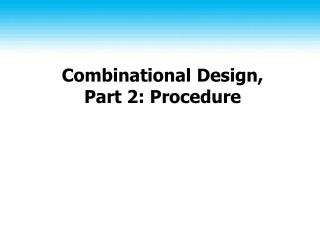DownloadDownload PresentationCombinational Design, Part 2: Procedure

# Combinational Design, Part 2: Procedure

Télécharger la présentation## Combinational Design, Part 2: Procedure

- - - - - - - - - - - - - - - - - - - - - - - - - - - E N D - - - - - - - - - - - - - - - - - - - - - - - - - - -
##### Presentation Transcript

1. Combinational Design,Part 2: Procedure

2. Topics • Positive vs. negative logic • Design procedure

3. Positive and Negative Logic Two different assignments of signal levels to logic values are possible • Using H level to represent a logic 1 (true) defines a positive logic system • Using H level to represent a logic 0 (true) defines a negative logic system

4. Assignment of Signal Levels to Logic Values

5. AND Gate Specification • IC data sheets define gates in terms of both signal values and logic values • Example: physical behavior specification of a CMOS gate • What is the logic function implemented by this gate ??

6. a polarity indicator: signify that NEGATIVE LOGIC is assumed for the signal Positive vs. Negative Logic

7. Bottom Line • Not much real change • Negative logic functions are just duals of positive logic ones • AND -> OR • OR -> AND

8. Design Procedure Similar to software • Specification – problem description • Write a specification for the circuit • Formulation – as truth table, Boolean function, or ( Verilog: in Lab) • define the required relationships between inputs and outputs • Optimization – used to be manual, now CAD tool • Draw a logic diagram or provide a netlist for the resulting circuit (AND, OR, INVERTORS) • Mapping – to select the implementation technology • Transform the diagram or netlist to a new diagram or netlist using the available implementation technology • Verification – used to be manual, now simulation • Verify correctness of design

9. 2. Formulation 3. Optimization 4. Mapping Example 1 • Specification:3 binary inputs; Output is 1 when input < 011

10. 5. Verification • Verify correctness of design • Manual: perform analysis of circuit • Simulation

11. Analysis of Circuit • Used in manual design verification and other purposes • This shows a disciplined way to analyze a circuit • To get Boolean function • and/or Truth table

12. Derivation of Func. Or Table • Label gate outputs in terms of “input variables” • Determine Boolean functions or values • Label outputs of gates fed by previously labeled gates • Determine Boolean function or values • Repeat 2 until done

13. Let’s Do This Example

14. Derivation of Truth Table • Make table with 2n rows, where n is number of inputs • Label some gate outputs • Put those labels and the final outputs on columns of truth table • Work your way across

15. Design Examples • Design Examples in the book • Study on your own: • Example 3-1 (p.99) : Design of a BCD-to-Excess-3 Converter • Example 3-2 (p.101) : Design of a BCD-Seven-Segment Decoder • Examples 3-3 (Will do in class) : Design of a 4-bit Equality Comparator • Input: 2 vectors A(3:0) and B(3:0) • Output: One bit, E, which is 1 if A and B are bitwise equal, 0 otherwise

16. Design of a 4-bit Equality Comparator • Specification : • Input: 2 vectors A(3:0) and B(3:0) • Output: One bit, E, which is 1 if A and B are bitwise equal, 0 otherwise • Hierarchical design seems a good approach • One module/bit • Final module for E

17. Design for MX module • Logic function Ei=0 if Ai and Bi have the same value; Ei = 1 if Ai and Bi have different values • Can implement as

18. Design for ME module • Final E is 1 only if all intermediate values are 0 • So • And a design is